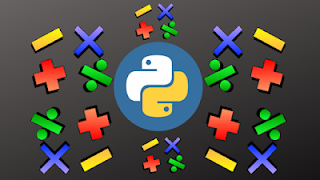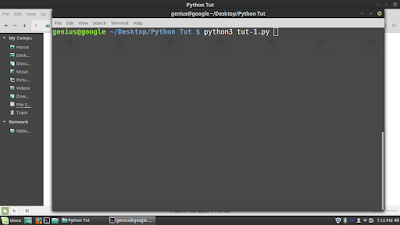# Python Program For Simple Calculator

0

In this tutorial i am going to write a python program for basic calculation such as additionsubtractionmultiplication and division using while loop. I hope it will be informative for you.Python Program For Simple Calculator

from os import system

while True:

system("clear")

print("Press 2: Subtract")

print("Press 3: Multiply")

print("Press 4: Divide")

print("Press 0: Exit")

if choice==1:

num1=eval(input("Enter a number:\n"))

num2=eval(input("Enter a number:\n"))

print("Sum = %g"%(num1+num2))

input("Press Enter")

elif choice==2:

num1=eval(input("Enter a number:\n"))

num2=eval(input("Enter a number:\n"))

print("Difference = %g"%(num1-num2))

input("Press Enter")

elif choice==3:

num1=eval(input("Enter a number:\n"))

num2=eval(input("Enter a number:\n"))

print("Product = %g"%(num1*num2))

input("Press Enter")

elif choice==4:

num1=eval(input("Enter a number:\n"))

num2=eval(input("Enter a number:\n"))

print("Quotient = %g"%(num1/num2))

input("Press Enter")

elif choice==0:

exit()

else:

print("Invalid Input\n")

input("Press Enter")

Save file as tut1.py

To run this program, open terminal and type: python3 tut1.pyChoose any one option by selecting one number and hit Enter. Here we select 3 for multiplication, you can select any number from 0 to 4.If you like the post or have any query don't hesitate, comment below....

Tags